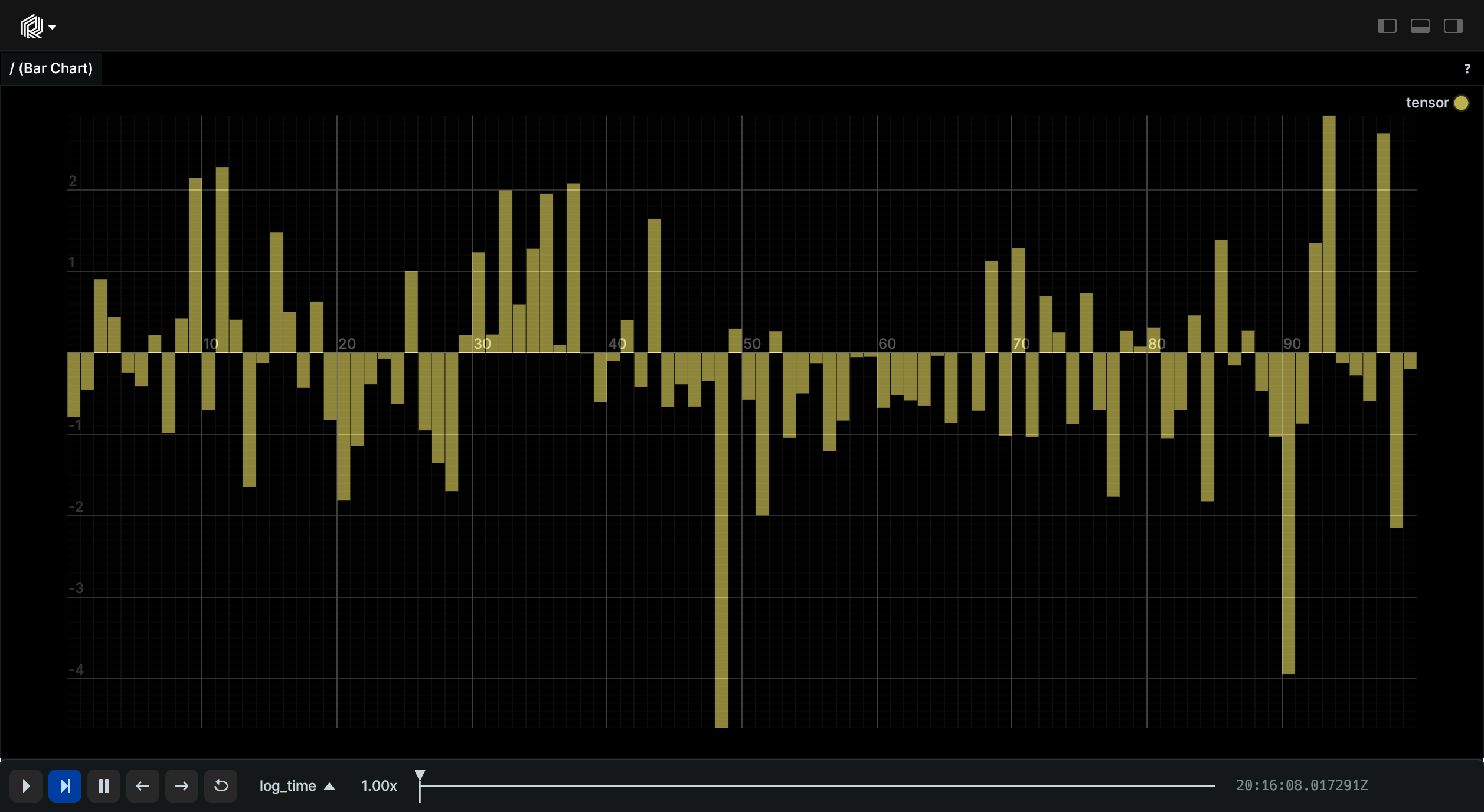# Tensor

Tensors are N-dimensional data matrix with homogeneous type. Supported types are:

• Unsigned integers: `uint8`, `uint16`, `uint32`, `uint64`
• Signed integers: `uint8`, `uint16`, `uint32`, `uint64`
• Floating point numbers: `float16`, `float32`, `float64`

Note: 1-D tensors are visualized as bar charts.

## Components and APIs

Primary component: `tensor`

Python APIs: log_tensor,

Rust API: Tensor

## Simple Example

``````"""Create and log a tensor."""

import rerun as rr
from numpy.random import default_rng

rng = default_rng(12345)

# Create a 4-dimensional tensor
tensor = rng.uniform(0.0, 1.0, (8, 6, 3, 5))

rr.init("rerun_example_tensors", spawn=True)

# Log the tensor, assigning names to each dimension
rr.log_tensor("tensor", tensor, names=("width", "height", "channel", "batch"))
``````## 1-D Tensor Example

``````"""Create and log a one dimensional tensor."""

import rerun as rr
from numpy.random import default_rng

rng = default_rng(12345)

# Create a 1-dimensional tensor
tensor = rng.laplace(0.0, 1.0, 100)

rr.init("rerun_example_tensors", spawn=True)

# Log the tensor, assigning names to each dimension
rr.log_tensor("tensor", tensor)
``````